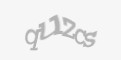## NUMBERS AND SCALE

The goal of the mathematics teaching project is to promote and understand the mathematical language that links hypotheses and observations, justifies predictions of real phenomena and indicates the relationships to be used in constructing mathematical models. The activities involve a multidisciplinary effective approach (with science and technology) in order to create a connection between mathematics and real life contexts through simple laboratory activities. Therefore, the result of calculation must be accompanied by checking and verification in the real life.

• Brief introduction to the practice :

The goal of this project is to provide students with experiential learning in the stages of preparing the material, analyzing and interpreting the data, and verifying the results.

Of course, we need to choose contexts and quantities to use, based on mathematical content. What we want is to emphasize and limit the proposal to phenomena that are simple to observe.

It is possible to use a scale to check the result of multiplication or division or, again with a scale, to check whether we have correctly calculated the weight of a given fraction of a small cake.

The project structure allows to include links and activities with other specific subjects in the school curriculum.

• Period of implementation: 10 Hours
• Country where the practice was developed : Italy
• Provider of the practice : APRO Formazione - Alba
• Targetg group: 15-19 y.o. students, EQF 3/4 level
• How to implement the practice :

The project can be carried out in several steps:

• - creation of groups consisting of 3 or 4 pupils
• - explanation of the project, which consists of performing simple arithmetic expressions whose results need to be verified with a classical weight scale
• - analysis and verification  of the results obtained
• - cenception and realization of  mathematical models to be adapted to the specific case
• - formalization through mathematical language  of the practical case study
• - public explanation of the used calculation methods and comparaison with other groups’ work
• Prerequisite knowledge:
• - Knowledge of natural, rational and integer numbers
• - Knowledge of arithmetic expressions
• - Knowledge of equivalences
• - Knowledge of ratios and proportions
• - Knowledge of the Cartesian plane
• - Knowledge of mathematical function
• Main learning outcomes from the practice :
• - Ability to work in a team
• - Ability to manage time
• - Promoting autonomy in study
• - Ability to observe and interpret the real-world context scientifically
• - Promote problem solving skills
• - Develop social skills
• - Using one's computer and digital skills
• - Presenting one's work in front of people
• - Developing imagination and creativity
• - Developing critical thinking
• - Developing individual and group communication
• Needed/Suggested digital tools:
• Suite Office or similar
• The practice was carried: : Hybrid online/classroom
• Critical points to shift towards STEM learning and solutions:

Students sometimes need their teacher help in many phases. The teacher in such a case should not provide solutions but help find solutions to all possible problems.

• Positive aspects of participatory learning :
• - Creation of a space for being together and sharing individual knowledge and skills
• - Cooperation among students
• - Each student can make his or her own contribution to achieve the goal
• - Lower-performing students can reach a higher level with the help of classmates and group work
• Negative aspects of participatory learning:

Management requires much versatility for the teacher and developed soft skills